Courses

# Analyzing Delay for Various Logic Circuits Electrical Engineering (EE) Notes | EduRev

## VLSI System Design

Created by: Machine Experts

## Electrical Engineering (EE) : Analyzing Delay for Various Logic Circuits Electrical Engineering (EE) Notes | EduRev

The document Analyzing Delay for Various Logic Circuits Electrical Engineering (EE) Notes | EduRev is a part of the Electrical Engineering (EE) Course VLSI System Design.
All you need of Electrical Engineering (EE) at this link: Electrical Engineering (EE)

Objectives

In this lecture you will learn the following

• Ratioed Logic
• Pass Transistor Logic
• Dynamic Logic Circuits

19.1 Ratioed Logic

Instead of combination of active pull down and pull up networks such a gate consists of an NMOS pull down network that realizes the logic function and a simple load device. For an inverter PDN is single NMOS transistor. The load can be a passive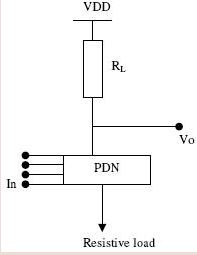Fig 19.1: Ratioed Logic Circuit

device, such as a resistor or an active element as a transistor. Let us assume that both PDN and load can be represented as linearized resistors. The operation is as follows: For a low input signal the pull down network is off and the output is high by the load. When the input goes high the driver transistor turns on , and the resulting output voltage is determined by the resistive division between the impedances of pull down and load network:

VOLRDVDD/(RD+RL)

where RD = pulldown n/w resistance, RL= load resistance.

To keep the low noise margin high it is important to chose RL>>RD . this style of logic
therefore called ratioed, because a careful PDN scaling of impedances(or transistor sizes) is required to obtain a workable gate. This is in contrast to the ratioless logic style as complementary CMOS, where the low and high level don’t depend upon transistor sizes. As a satisfactory level we keep RL>=4RD . To achieve this, (W/L)D/(W/L)L> 4.

19.2 Pass Transistor Logic

The fundamental building block of nMOS dynamic logic circuit, consisting of an nMOS pass transistor is shown in figure 19.21.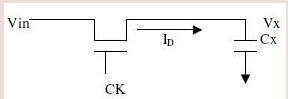Fig 19.21: Pass Transistor Logic Circuit

The pass transistor MP is driven by the periodic clock signal and acts as an access switch to either charge up or down the parasitic capacitance, Cx, depending on the input signal Vin. Thus there are 2 possible operations when the clock signal is active are the logic “1” transfer( charging up the capacitance Cx to logic high level) and the logic “0” transfer( charging down the capacitance Cx to a logic low level). In either case, the output of  the depletion load of the nMOS inverter obviously assumes a logic low or high level, depending on the voltage Vx.

The pass transistor MP provides the only current path to the intermediate capacitive node X. when clock signal becomes inactive (clk=0) the pass transistor ceases to conduct and the charge is stored in the parasitic capacitor Cx continues to determine the output level of the inverter.

Logic “1” Transfer: Assume that the Vx = 0 initially. A logic "1"level is applied to the input terminal which corresponds to Vin=VOH=VDD. Now the clock signal at the gate of the pass transistor goes from 0 to VDD at t=0. It can be seen that the pass
transistor starts to conduct and operate in saturation throughout this cycle since VDS=VGS. Consequently VDS> VGS-Vtn.

Analysis: The pass transistor operating in saturation region starts to charge up the capacitor Cx, thus: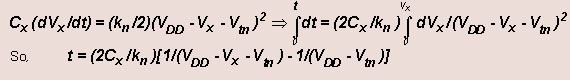The previous equation for Vx(t) can be solved as-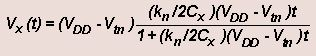The variation of the node voltage Vx(t)is plotted as a function of time in fig. 19.22. The voltage rises from its initial value of 0 and reaches Vmax =VDD-Vtn after a large time. The pass transistor will turn off when Vx = Vmax. Since Vgs= Vtn. Therefore Vx can never attain VDD during logic 1 transfer. Thus we can use buffering to overcome this problem.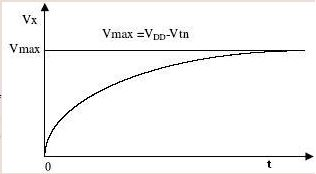Fig 19.22: Node Voltage Vx vs t

Logic “0” Transfer: Assume that the Vx=1 initially. A logic“0” level is  applied to the input terminal which corresponds to Vin=1. Now the clock signal at the gate of the pass transistor goes from 0 toVDD at t=0. It can be seen that the pass transistor starts to conduct and operate in linear mode throughout this cycle and the drain current flows in the opposite direction to that of charge up.

Analysis: We can write -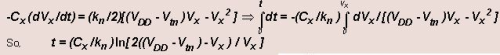The above equation for Vx(t) can be solved as -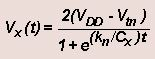Plot of Vx(t) is shown in figure 19.23.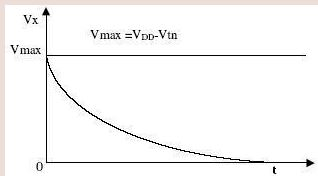Fig 19.22: Node Voltage Vx vs t

19.3 Dynamic Logic Circuits

In case of static CMOS for a fan-in of N2N transistors are required. In order to reduce this, various other design logics were used like pseudo-NMOS logic and pass transistor logic. However the static power consumption in these cases increased. An alternative to these design logics is Dynamic logic, which reduces the number of transistors at the same time keeps a check on the static power consumption.

Principle: A block diagram of a dynamic logic circuit is as shown in fig 19.31. This uses NMOS block to implement its logic

The operation of this circuit can be explained in two modes.
1) Precharge
2) Evaluation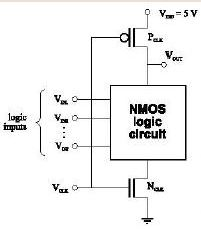Fig 19.31: Dynamic CMOS Block Diagram

In the precharge mode, the CLK input is at logic 0. This forces the output to logic 1, charging the load capacitance to VDD. Since the NMOS transistor M1 is off the pull-down
path is disabled. There is no static consumption in this case as there is no direct path
between supply and ground.

In the evaluation mode, the CLK input is at logic 1. Now the output depends on the PDN block. If there exists a path through PDN to ground (i.e. the PDN network is ON), the capacitor CL will discharge else it remains at logic 1.As there exists only one path between the output node and a supply rail, which can only be ground, the load capacitor can discharge only once and if this happens, it cannot charge until the next precharge operation. Hence the inputs to the gate can make at most one transition during evaluation

1) As can be seen, the number of transistors required here are N+2 as compared to 2N in the Static CMOS circuits.

2) This circuit is still a ratioless circuit as in Static case. Hence, progressive sizing and ordering of the transistors in the PDN block is important.
3) As can be seen, the static power loss is negligible.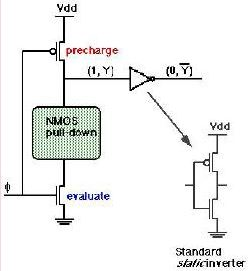19.32: DOMINO CMOS Block Diagram

1) The penalty paid in such circuits is that the clock must run everywhere to each such block as shown in the diagram.
2) The major problem in such circuits is that the output node is at Vdd till the end of the precharge mode. Now if the CLK in the next block arrives earlier compared to the CLK in this block, or the PDN network in this block takes a longer time to evaluate its output, then the next block will start to evaluate using this erroneous value

The second part of the disadvantage can be eliminated by using DOMINOCMOS circuits which are as shown below.

As can be seen the output at the end of precharge is inverted by the inverter to logic 0. Thus the next block will not be evaluated till this output has been evaluated. As an ending point, it must be noted that this also has a disadvantage that since at each stage the output is inverted, the logic must be changed to accommodate this.

Offer running on EduRev: Apply code STAYHOME200 to get INR 200 off on our premium plan EduRev Infinity!

35 docs|28 tests

,

,

,

,

,

,

,

,

,

,

,

,

,

,

,

,

,

,

,

,

,

;